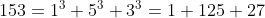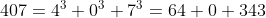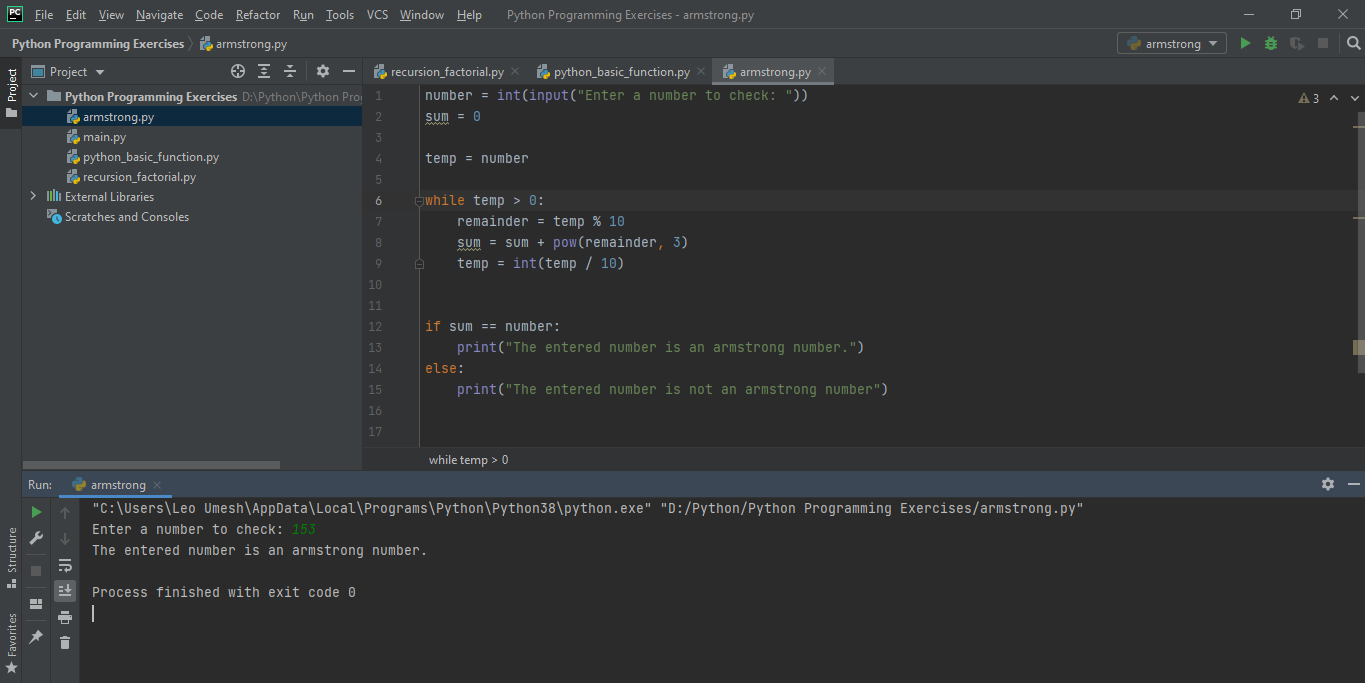# PYTHON PROGRAMMING EXERCISES 2: CHECKING WHETHER A GIVEN NUMBER IS ARMSTRONG OR NOT

in STEMGeeks5 months ago

Following is a simple python program to check whether a user entered number is an Armstrong number or not. Before going into the code, let me tell you what Armstrong numbers are. They are the numbers whose sum of cube of individual digits is equal to the number itself. For example:``````number = int(input("Enter a number to check: "))
sum = 0

temp = number

while temp > 0:
remainder = temp % 10
sum = sum + pow(remainder, 3)
temp = int(temp / 10)

if sum == number:
print("The entered number is an armstrong number.")
else:
print("The entered number is not an armstrong number")
``````

The program will prompt user to enter a number and store it in a temporary variable called temp. We will initialize a sum variable to 0. And do our task of completing the sum inside while loop. When the loop terminates, then it will compare the sum to our original number and it is equal then it is an Armstrong number else not. Lets see the output of above code: